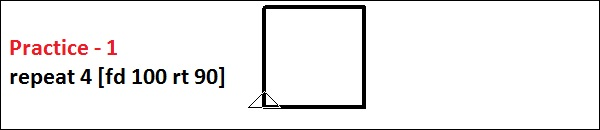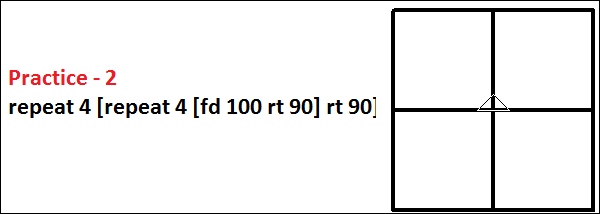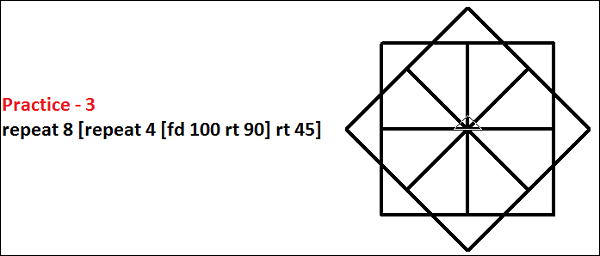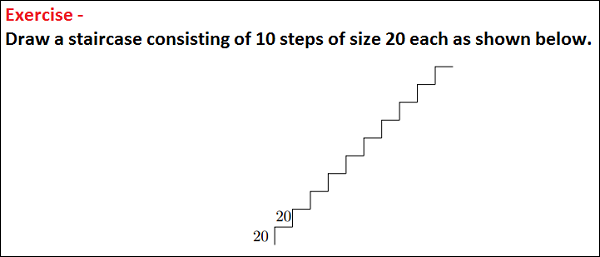# Logo - Repetition

We often repeat a sequence of commands. Computer programs often perform repetitive tasks. Just about every programming system has a way of carrying out this repetition, or iteration, as computer scientists call it. Let us consider the following example −

Let us assume we want to draw a square with sides of length 100, we can do this with the following program −

```fd 100
rt 90
fd 100
rt 90
fd 100
rt 90
fd 100
rt 90
```

We note that the two commands – fd 100 and rt 90 are repeated four times. Will it not be simpler to tell the computer that it should just repeat these two commands four times instead of writing them four times in a row? We can do exactly this, using the following command −It saves our time of typing-in to make a square. The general form is: repeat number [commands]. We must use the keyword – repeat followed by a number and then a sequence of commands in [square brackets].

Often, we might have to repeat within repeat. This is called nesting. Let us look at some examples on this.Following is an exercise to check your aptitude on what you have learnt so far in this chapter.